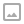## Exploring Dot Products in Unity

·   ·  ☕ 2 min read

Dot Products are a really easy and fun way to compare Vectors in space. When comparing two normalized with a dot product the result will be the cosine of the angle between the two Vectors. This means that for normalized Vectors the results will be:

AlignmentResult
Parallel1
Anti-Parallel-1
Orthogonal0

Taking the ArcCosine of these values will return the angle between the two Vectors (Note that Unity uses radians for most calculations so you may need to convert to degrees yourself).

Lets explore some applications of Dot Products and show off how to take advantage of them in your games or other projects. For example, how can you detect if a point is inside of a cone? Perhaps as a way to check if an enemy AI can see the player or another reason. A second example may be detecting the difference in orientation between joysticks and a vehicles orientation to detect if a turn or thrust is the appropriate action to perform when moving.

The formula for dot products is the sum of the product of each axis. For example the dot product of two vectors A and B would be:

• In 2D space: (A.x * B.x) + (A.y * B.y)
• In 3D space: (A.x * B.x) + (A.y * B.y) + (A.z * B.z)
• In 4D space: (A.x * B.x) + (A.y * B.y) + (A.z * B.z) + (A.w * B.w)
• This formula can be expanded as the dimensions continues to increase if you need it to.

Note: Because of this formula the length of the Vectors being compared in the dot product does matter!

A similar application of dot products but in shader form in our revealing flashlight shader: https://youtu.be/b4utgRuIekk

An example of using dot products to help control how a top down spaceship uses it’s thrusters and rotation: https://youtu.be/TUIHY-5MvWQ

Source Code for this series is available on GitHub: https://github.com/WorldOfZero/top-down-spaceship

Join the World of Zero Discord server: https://discord.gg/hU5Kq2uWRITTEN BY
Sam Wronski
Maker of things and professional software engineer. Lets make something awesome together!